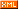posts - 21, comments - 461, trackbacks - 0, articles - 0
::  ::  ::  ::::
 前言：

Sencha Touch 交流 QQ 群 213119459 欢迎您的加入。

 本节目标：加载 ST2 到你的网页，搭建运行环境

ST1的时候，搭建基于ST的网页运行环境的确非常简单，如官方这篇文档所述已经足够（这也是我认为诚意不够的原因，为嘛不紧跟ST2的步伐呢）。

 开发环境说明

 Demo代码完整结构SenchaTouch文件夹下面的2.0.0文件夹表明当前ST框架的版本号，再下面就是ST2框架的所有相关资源文件；

• 以sencha-touch开头的4个js文件分别是ST2框架的all版和core版及其各自的debug版；
• css里面放置了ST2的几套皮肤样式文件；
• src文件夹下是ST2的所有源文件代码，该文件夹内文件主要供core加载方式调用；

Demo文件夹下就是我们此次编写的全部代码文件：

 Demo所实现的效果：文本框与Picker结合实例A.直接加载完整框架文件（all方式）

sencha-touch-all.html代码:

<!DOCTYPE html><html><head>    <title>Hello Mobile</title>    <link rel="stylesheet" href="http://www.cnblogs.com/SenchaTouch/2.0.0/css/sencha-touch.css" type="text/css">    <script type="text/javascript" src="http://www.cnblogs.com/SenchaTouch/2.0.0/sencha-touch-all-debug.js"></script>    <script type="text/javascript" src="app-all.js"></script></head><body></body></html>

app-all.js代码（代码逻辑很简单，看注释即可）：

Ext.application({    name: 'Demo',    viewport: {        autoMaximize: true // 该属性可以设置页面自动最大化（隐藏地址栏）    },    launch: function () {        Ext.create("Ext.Panel", {            fullscreen: true,            items: [                {                    xtype: 'fieldset',                    margin: 10,                    title: '文本框与Picker结合实例',                    items: [                        {                            xtype: 'textfield',                            name: 'aTextField',                            id: 'aTextField',                            readOnly: true,         // 把文本框设为只读，禁止输入                            label: '取值结果',                            clearIcon: true,                            listeners: {                                // 侦听文本框的focus事件，获取到焦点时触发                                focus: function () {                                    this.disable();                 // 先禁用文本框，防止系统调出软键盘                                    Ext.getCmp('aPicker').show();   // 然后显示用来选择内容的Picker                                }                            }                        }                    ]                }            ]        });        // 定义一个Picker供文本框联动        aPicker = Ext.create('Ext.Picker', {            name: 'aPicker',            id: 'aPicker',            hidden: true,            listeners: {                // 侦听change事件，Picker的值改变同时也设定文本框的值                change: function () {                    Ext.getCmp('aTextField').setValue(aPicker.getValue().question);                },                // 侦听hide事件，当Picker消失时将文本框状态恢复为enable                hide: function () {                    Ext.getCmp('aTextField').enable();                }            },            slots: [                {                    name: 'question',                    data: [                        {                            text: '无',                            value: ''                        },                        {                            text: '最喜欢的颜色',                            value: 'color'                        },                        {                            text: '最喜欢的运动',                            value: 'sport'                        },                        {                            text: '最喜欢的明星',                            value: 'star'                        }                    ]                }            ]        });        // 前面定义的Picker控件必须显式加入Viewport，否则无法被调用显示        // Ext.Viewport是Sencha Touch自动创建的一个顶级容器        Ext.Viewport.add(aPicker);    }});

 B.先加载核心框架文件，然后按需加载其他组件（core方式）

• 单页面加载文件的个数大大增加，这毫无疑问会加大服务器的并发压力；
• 按需加载方式还将导致页面渲染时间（指页面所需文件下载完成到页面渲染完成中间这段页面显示白屏的时长）变长，用户体验未见明显提升。

sencha-touch-core.html代码（相比A所采用的all方式，仅仅改变了对应用程序代码文件（app-core.js取代了app-all.js）和框架js文件（sencha-touch-debug.js文件取代sencha-touch-all-debug.js文件）的引用：

<!DOCTYPE html><html><head>    <title>Hello Mobile</title>    <link rel="stylesheet" href="http://www.cnblogs.com/SenchaTouch/2.0.0/css/sencha-touch.css" type="text/css">    <script type="text/javascript" src="http://www.cnblogs.com/SenchaTouch/2.0.0/sencha-touch-all-debug.js"></script>    <script type="text/javascript" src="app-core.js"></script></head><body></body></html>

app-core.js代码（着重观察开始的部分，后面的代码与all方式没有区别）：

// 第一件事就是给src这个源文件目录指定一个命名空间（具体参见官方文档翻译中的External Dependencies，即外部依赖）Ext.Loader.setPath({    'Ext': 'http://www.cnblogs.com/SenchaTouch/2.0.0/src'});// 然后根据application用到的控件来添加对以下组件的引用（有人说Ext.TitleBar没用到啊，其实用到了，在Picker里面）// 大家请注意，其实这里的Ext命名空间指的就是上面Ext.Loader.setPath方法中指定的外部依赖命名空间Ext.require(['Ext.form.FieldSet', 'Ext.picker.Picker', 'Ext.TitleBar']);// 下面的代码部分跟all方式完全保持了一致，故省略……Ext.application({    name: 'Demo',    ……

<!DOCTYPE HTML><html><head>    <meta charset="UTF-8">    <title>Microloader</title>    <script id="microloader" type="text/javascript" src="http://www.cnblogs.com/SenchaTouch/2.0.0/microloader/development.js"></script></head><body></body></html>

{    "name": "Demo",  // app名称    "js": [        {            "path": "http://www.cnblogs.com/SenchaTouch/2.0.0/sencha-touch-all-debug.js"  // 引用的ST框架文件，这里我们选用all模式        },        {            "path": "app.js",  // 引用的应用程序代码文件            "update": "delta"        }    ],    "css": [        {            "path": "http://www.cnblogs.com/SenchaTouch/2.0.0/css/sencha-touch.css",  // 引用的css文件            "update": "delta"        }    ],    "appCache": {        "cache": [            "JsCodeBehind.html",            "JsCodeInline.html"        ],        "network": [            "*"        ],        "fallback": []    },    "extras": [    ],    "archivePath": "archive",    "buildPaths": {        "testing": "http://www.cnblogs.com/deploy/testing/Demo",        "production": "http://www.cnblogs.com/deploy/production/Demo",        "package": "http://www.cnblogs.com/deploy/package/Demo",        "native": "http://www.cnblogs.com/deploy/native/Demo"    },    "buildOptions": {        "product": "touch",        "minVersion": 3,        "debug": false,        "logger": "no"    },    "id": "3a867610-670a-11e1-a90e-4318029d18bb"}

Code-inline方式，顾名思义，把js代码直接写在html文件里，这是目前ST官网实例中所采用的方式（您可能已经注意到了，官方的例子与提供下载的sdk包中同一个例子所采取的方式并不相同）！

<!DOCTYPE HTML><html manifest="cache.manifest" lang="en-US"><head>    <meta charset="UTF-8">    <title>TouchStyle</title>    <script type="text/javascript">(function(n){function r(a){function b(a,j){var c=a.length,b,e;for(b=0;b<c;b++){e=a[b];var d=a,J=b,k=void 0;"string"==typeof e&&(e={path:e});e.shared?(e.version=e.shared,k=e.shared+e.path):(y.href=e.path,k=y.href);e.uri=k;e.key=g+"-"+k;f[k]=e;d[J]=e;e.type=j;e.index=b;e.collection=a;e.ready=!1;e.evaluated=!1}return a}var c;"string"==typeof a?(c=a,a=z(c)):c=JSON.stringify(a);var g=a.id,d=g+"-"+A+o,f={};this.key=d;this.css=b(a.css,"css");this.js=b(a.js,"js");this.assets=this.css.concat(this.js);this.getAsset=function(a){return f[a]};this.store=function(){s(d,c)}}function v(a,b){i.write('<meta name="'+a+'" content="'+b+'">')}function p(a,b,c){var g=new XMLHttpRequest,d,c=c||B;try{g.open("GET",a,!0),g.onreadystatechange=function(){4==g.readyState&&(d=g.status,200==d||304==d||0==d?b(g.responseText):c())},g.send(null)}catch(f){c()}}function K(a,b){var c=i.createElement("iframe");m.push({iframe:c,callback:b});c.src=a+".html";c.style.cssText="width:0;height:0;border:0;position:absolute;z-index:-999;visibility:hidden";i.body.appendChild(c)}function C(a,b,c){var g=!!a.shared;if(!g)var d=b,f=a.version,h,b=function(j){h=j.substring(0,f.length+4);h!=="/*"+f+"*/"?confirm("Requested: '"+a.uri+"' with checksum: "+f+" but received: "+h.substring(2,f.length)+"instead. Attemp to refresh the application?")&&L():d(j)};(g?K:p)(a.uri,b,c)}function D(a){var b=a.data,a=a.source.window,c,g,d,f;for(c=0,g=m.length;c<g;c++)if(d=m[c],f=d.iframe,f.contentWindow===a){d.callback(b);i.body.removeChild(f);m.splice(c,1);break}}function E(a){"undefined"!=typeof console&&(console.error||console.log).call(console,a)}function s(a,b){try{l.setItem(a,b)}catch(c){if(c.code==c.QUOTA_EXCEEDED_ERR){var g=t.assets.map(function(a){return a.key}),d=0,f=l.length,h=!1,j;for(g.push(t.key);d<=f-1;)j=l.key(d),-1==g.indexOf(j)?(l.removeItem(j),h=!0,f--):d++;h&&s(a,b)}}}function u(a){try{return l.getItem(a)}catch(b){return null}}function L(){F||(F=!0,p(o,function(a){(new r(a)).store();n.location.reload()}))}function G(a){function b(a,b){var d=a.collection,e=a.index,g=d.length,f;a.ready=!0;a.content=b;for(f=e-1;0<=f;f--)if(a=d[f],!a.ready||!a.evaluated)return;for(f=e;f<g;f++)if(a=d[f],a.ready)a.evaluated||c(a);else break}function c(a){a.evaluated=!0;if("js"==a.type)try{eval(a.content)}catch(b){E("Error evaluating "+a.uri+" with message: "+b)}else{var c=i.createElement("style"),e;c.type="text/css";c.textContent=a.content;"id"in a&&(c.id=a.id);"disabled"in a&&(c.disabled=a.disabled);e=document.createElement("base");e.href=a.path.replace(/\/[^\/]*\$/,"/");w.appendChild(e);w.appendChild(c);w.removeChild(e)}delete a.content;0==--f&&g()}function g(){function c(){d&&b()}function b(){var a=q.onUpdated||B;if("onSetup"in q)q.onSetup(a);else a()}function f(){l.store();e.forEach(function(a){s(a.key,a.content)});b()}var e=[],d=!1,g=!1,k=function(){g=!0},i=function(){h.swapCache();d=!0;k()},m;n.removeEventListener("message",D,!1);h.status==h.UPDATEREADY?i():h.status==h.CHECKING||h.status==h.DOWNLOADING?(h.onupdateready=i,h.onnoupdate=h.onobsolete=k):k();!1!==navigator.onLine&&p(o,function(b){t=l=new r(b);var d;l.assets.forEach(function(b){d=a.getAsset(b.uri);(!d||b.version!==d.version)&&e.push(b)});m=e.length;0==m?g?c():k=c:e.forEach(function(b){function c(){C(b,function(a){b.content=a;0==--m&&(g?f():k=f)})}var d=a.getAsset(b.uri),e=b.path,h=b.update;!d||!h||null===u(b.key)||"delta"!=h?c():p("deltas/"+e+"/"+d.version+".json",function(a){try{var c=b,d;var e=u(b.key),h=z(a),a=[],j,i,l;if(0===h.length)d=e;else{for(i=0,l=h.length;i<l;i++)j=h[i],"number"===typeof j?a.push(e.substring(j,j+h[++i])):a.push(j);d=a.join("")}c.content=d;0==--m&&(g?f():k=f)}catch(n){E("Malformed delta content received for "+b.uri)}},c)})})}var d=a.assets,f=d.length,l;t=a;H("message",D,!1);0==f?g():d.forEach(function(a){var c=u(a.key);null===c?C(a,function(c){s(a.key,c);b(a,c)},function(){b(a,"")}):b(a,c)})}function I(a){null!==i.readyState.match(/interactive|complete|loaded/)?G(a):H("DOMContentLoaded",function(){G(a)},!1)}var B=function(){},m=[],i=n.document,w=i.head,H=n.addEventListener,l=n.localStorage,h=n.applicationCache,z=JSON.parse,y=i.createElement("a"),x=i.location,A=x.origin+x.pathname+x.search,o="app.json",F=!1,t;if("undefined"===typeof q)var q=n.Ext={};q.blink=function(a){var b=u(a.id+"-"+A+o);v("viewport","width=device-width, initial-scale=1.0, maximum-scale=1.0, minimum-scale=1.0, user-scalable=no");v("apple-mobile-web-app-capable","yes");v("apple-touch-fullscreen","yes");b?(a=new r(b),I(a)):p(o,function(b){a=new r(b);a.store();I(a)})}})(this);;Ext.blink({"id":"3a867610-670a-11e1-a90e-4318029d18bb"})</script></head><body></body></html>

<!DOCTYPE HTML><html><head>    <meta charset="UTF-8">    <title>Microloader</title>    <script type="text/javascript">        (function () {            function write(content) {                document.write(content);            }            function meta(name, content) {                write('<meta name="' + name + '" content="' + content + '">');            }            var xhr = new XMLHttpRequest();            xhr.open('GET', 'app.json', false);            xhr.send(null);            var options = eval("(" + xhr.responseText + ")"), scripts = options.js || [], styleSheets = options.css || [], i, ln, path;            meta('viewport', 'width=device-width, initial-scale=1.0, maximum-scale=1.0, minimum-scale=1.0, user-scalable=no');            meta('apple-mobile-web-app-capable', 'yes');            meta('apple-touch-fullscreen', 'yes');            for (i = 0, ln = styleSheets.length; i < ln; i++) {                path = styleSheets[i];                if (typeof path != 'string') {                    path = path.path;                }                write('<link rel="stylesheet" href="' + path + '">');            }            for (i = 0, ln = scripts.length; i < ln; i++) {                path = scripts[i];                if (typeof path != 'string') {                    path = path.path;                }                write('<script src="' + path + '"></' + 'script>');            }        })();    </script></head><body></body></html>

 结语：

Sencha Touch 交流 QQ 群 213119459 欢迎您的加入。